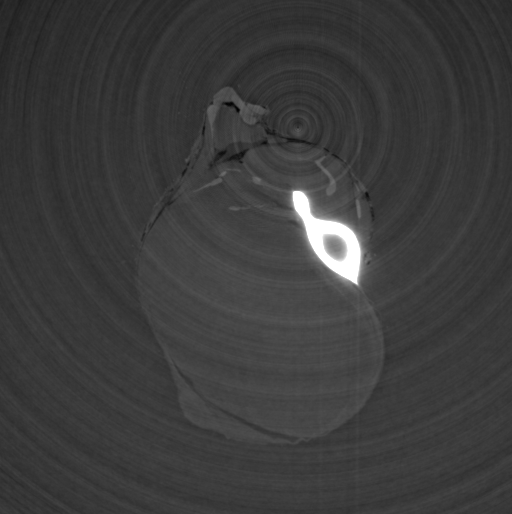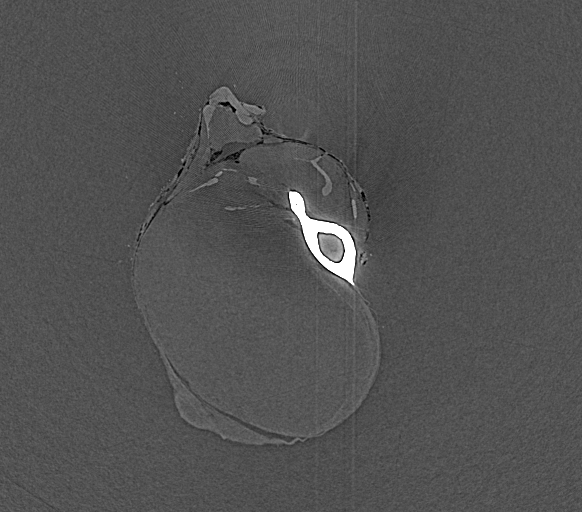# Ring Correction with Thresholding¶

You find the the example in

/scisoft/ESRF_sw/TEST_PYHST/SINO_THRESHOLDInput File:

# HST_SLAVE PARAMETER FILE

OUTPUT_FILE = nocorr.vol

NUM_FIRST_IMAGE = 0 # No. of first projection file
NUM_LAST_IMAGE = 1199 # No. of last projection file
NUMBER_LENGTH_VARIES = NO
LENGTH_OF_NUMERICAL_PART = 4 # No. of characters
FILE_POSTFIX = .edf
FILE_INTERVAL = 1 # Interval between input files
# Parameters defining the projection file format

NUM_IMAGE_1 = 1300 # Number of pixels horizontally
NUM_IMAGE_2 = 980 # Number of pixels vertically

IMAGE_PIXEL_SIZE_1 = 46.6000 # Pixel size horizontally (microns)
IMAGE_PIXEL_SIZE_2 = 46.6000 # Pixel size vertically

# Parameters defining background treatment
SUBTRACT_BACKGROUND = YES # Subtract background from data
# Parameters defining flat-field treatment
CORRECT_FLATFIELD = YES # Divide by flat-field image
FLATFIELD_CHANGING = NO # Series of flat-field files
TAKE_LOGARITHM = YES # Take log of projection values
# Parameters defining experiment

ANGLE_BETWEEN_PROJECTIONS = 0.15 # Increment angle in degrees

ROTATION_VERTICAL = YES
ROTATION_AXIS_POSITION =  883-150 # Position in pixels

START_VOXEL_1 =      1 # X-start of reconstruction volume
START_VOXEL_2 =      1 # Y-start of reconstruction volume
START_VOXEL_3 =   122 # Z-start of reconstruction volume
END_VOXEL_1 =   1300 # X-end of reconstruction volume
END_VOXEL_2 =   1300# Y-end of reconstruction volume
END_VOXEL_3 =   122 # Z-end of reconstruction volume

OVERSAMPLING_FACTOR = 4 # 0 = Linear, 1 = Nearest pixel
ANGLE_OFFSET = 0.000000 # Reconstruction rotation offset angle in degrees
CACHE_KILOBYTES = 4096 # Size of processor cache (L2) per processor (KBytes)
SINOGRAM_MEGABYTES = 400 # Maximum size of sinogram storage (megabytes)
# Parameters extra features PyHST
DO_CCD_FILTER = NO # CCD filter (spike
CCD_FILTER = "CCD_Filter"
CCD_FILTER_PARA =  {"threshold": 0.040000 }

DO_RING_FILTER = NO # Sinogram filter (rings)
RING_FILTER = "RING_Filter"
ar = Numeric.ones(1300,'f')
ar=0.0
RING_FILTER_PARA = {"FILTER": ar, "threshold":0.15 }

DO_AXIS_CORRECTION = NO # Axis correction
AXIS_CORRECTION_FILE = correct.txt

OPTIONS= { 'padding':'E' , 'axis_to_the_center':'Y' , 'avoidhalftomo':'Y'} # Padding and position axis

# Parameters for Paganin reconstruction
# delta over beta ratio for Paganin phase retrieval = 200.00
DO_PAGANIN = 1
PAGANIN_Lmicron = 1050
PAGANIN_MARGE = 20
DO_OUTPUT_PAGANIN = 0
OUTPUT_PAGANIN_FILE = paga_cufft
OUTPUT_PAGANIN_START=0
OUTPUT_PAGANIN_END  =20
PAGANIN_TRY_CUFFT = 1
PAGANIN_TRY_FFTW = 1
UNSHARP_LOG = 1
PUS = 0.000000
PUC = 2.000000

PENTEZONE=300
ZEROCLIPVALUE = 0.0000000001 # Minimum value of radiographs after flat / before log

FBFILTER=1Input File:

# HST_SLAVE PARAMETER FILE

OUTPUT_FILE = ringcorr.vol

NUM_FIRST_IMAGE = 0 # No. of first projection file
NUM_LAST_IMAGE = 1199 # No. of last projection file
NUMBER_LENGTH_VARIES = NO
LENGTH_OF_NUMERICAL_PART = 4 # No. of characters
FILE_POSTFIX = .edf
FILE_INTERVAL = 1 # Interval between input files
# Parameters defining the projection file format

NUM_IMAGE_1 = 1300 # Number of pixels horizontally
NUM_IMAGE_2 = 980 # Number of pixels vertically

IMAGE_PIXEL_SIZE_1 = 46.6000 # Pixel size horizontally (microns)
IMAGE_PIXEL_SIZE_2 = 46.6000 # Pixel size vertically

# Parameters defining background treatment
SUBTRACT_BACKGROUND = YES # Subtract background from data
# Parameters defining flat-field treatment
CORRECT_FLATFIELD = YES # Divide by flat-field image
FLATFIELD_CHANGING = NO # Series of flat-field files
TAKE_LOGARITHM = YES # Take log of projection values
# Parameters defining experiment

ANGLE_BETWEEN_PROJECTIONS = 0.15 # Increment angle in degrees

ROTATION_VERTICAL = YES
ROTATION_AXIS_POSITION =  883-150 # Position in pixels

START_VOXEL_1 =      1 # X-start of reconstruction volume
START_VOXEL_2 =      1 # Y-start of reconstruction volume
START_VOXEL_3 =   122 # Z-start of reconstruction volume
END_VOXEL_1 =   1300 # X-end of reconstruction volume
END_VOXEL_2 =   1300# Y-end of reconstruction volume
END_VOXEL_3 =   122 # Z-end of reconstruction volume

OVERSAMPLING_FACTOR = 4 # 0 = Linear, 1 = Nearest pixel
ANGLE_OFFSET = 0.000000 # Reconstruction rotation offset angle in degrees
CACHE_KILOBYTES = 4096 # Size of processor cache (L2) per processor (KBytes)
SINOGRAM_MEGABYTES = 400 # Maximum size of sinogram storage (megabytes)
# Parameters extra features PyHST
DO_CCD_FILTER = NO # CCD filter (spike
CCD_FILTER = "CCD_Filter"
CCD_FILTER_PARA =  {"threshold": 0.040000 }

DO_RING_FILTER = YES # Sinogram filter (rings)
RING_FILTER = "RING_Filter"
ar = Numeric.ones(1300,'f')
ar=0.0
RING_FILTER_PARA = {"FILTER": ar, "threshold":0.15 }

DO_AXIS_CORRECTION = NO # Axis correction
AXIS_CORRECTION_FILE = correct.txt

OPTIONS= { 'padding':'E' , 'axis_to_the_center':'Y' , 'avoidhalftomo':'Y'} # Padding and position axis

# Parameters for Paganin reconstruction
# delta over beta ratio for Paganin phase retrieval = 200.00
DO_PAGANIN = 1
PAGANIN_Lmicron = 1050
PAGANIN_MARGE = 20
DO_OUTPUT_PAGANIN = 0
OUTPUT_PAGANIN_FILE = paga_cufft
OUTPUT_PAGANIN_START=0
OUTPUT_PAGANIN_END  =20
PAGANIN_TRY_CUFFT = 1
PAGANIN_TRY_FFTW = 1
UNSHARP_LOG = 1
PUS = 0.000000
PUC = 2.000000

PENTEZONE=300
ZEROCLIPVALUE = 0.0000000001 # Minimum value of radiographs after flat / before log

FBFILTER=1Input File:

# HST_SLAVE PARAMETER FILE

OUTPUT_FILE = threshold.vol

NUM_FIRST_IMAGE = 0 # No. of first projection file
NUM_LAST_IMAGE = 1199 # No. of last projection file
NUMBER_LENGTH_VARIES = NO
LENGTH_OF_NUMERICAL_PART = 4 # No. of characters
FILE_POSTFIX = .edf
FILE_INTERVAL = 1 # Interval between input files
# Parameters defining the projection file format

NUM_IMAGE_1 = 1300 # Number of pixels horizontally
NUM_IMAGE_2 = 980 # Number of pixels vertically

IMAGE_PIXEL_SIZE_1 = 46.6000 # Pixel size horizontally (microns)
IMAGE_PIXEL_SIZE_2 = 46.6000 # Pixel size vertically

# Parameters defining background treatment
SUBTRACT_BACKGROUND = YES # Subtract background from data
# Parameters defining flat-field treatment
CORRECT_FLATFIELD = YES # Divide by flat-field image
FLATFIELD_CHANGING = NO # Series of flat-field files
TAKE_LOGARITHM = YES # Take log of projection values
# Parameters defining experiment

ANGLE_BETWEEN_PROJECTIONS = 0.15 # Increment angle in degrees

ROTATION_VERTICAL = YES
ROTATION_AXIS_POSITION =  883-150 # Position in pixels

START_VOXEL_1 =      1 # X-start of reconstruction volume
START_VOXEL_2 =      1 # Y-start of reconstruction volume
START_VOXEL_3 =   122 # Z-start of reconstruction volume
END_VOXEL_1 =   1300 # X-end of reconstruction volume
END_VOXEL_2 =   1300# Y-end of reconstruction volume
END_VOXEL_3 =   122 # Z-end of reconstruction volume

OVERSAMPLING_FACTOR = 4 # 0 = Linear, 1 = Nearest pixel
ANGLE_OFFSET = 0.000000 # Reconstruction rotation offset angle in degrees
CACHE_KILOBYTES = 4096 # Size of processor cache (L2) per processor (KBytes)
SINOGRAM_MEGABYTES = 400 # Maximum size of sinogram storage (megabytes)
# Parameters extra features PyHST
DO_CCD_FILTER = NO # CCD filter (spike
CCD_FILTER = "CCD_Filter"
CCD_FILTER_PARA =  {"threshold": 0.040000 }

DO_RING_FILTER = YES # Sinogram filter (rings)
RING_FILTER = "RING_Filter_THRESHOLDED"
ar = Numeric.ones(1300,'f')
ar=0.0
RING_FILTER_PARA = {"FILTER": ar, "threshold":0.15 }

DO_AXIS_CORRECTION = NO # Axis correction
AXIS_CORRECTION_FILE = correct.txt

OPTIONS= { 'padding':'E' , 'axis_to_the_center':'Y' , 'avoidhalftomo':'Y'} # Padding and position axis

# Parameters for Paganin reconstruction
# delta over beta ratio for Paganin phase retrieval = 200.00
DO_PAGANIN = 1
PAGANIN_Lmicron = 1050
PAGANIN_MARGE = 20
DO_OUTPUT_PAGANIN = 0
OUTPUT_PAGANIN_FILE = paga_cufft
OUTPUT_PAGANIN_START=0
OUTPUT_PAGANIN_END  =20
PAGANIN_TRY_CUFFT = 1
PAGANIN_TRY_FFTW = 1
UNSHARP_LOG = 1
PUS = 0.000000
PUC = 2.000000

PENTEZONE=300
ZEROCLIPVALUE = 0.0000000001 # Minimum value of radiographs after flat / before log

FBFILTER=1Input File:

# HST_SLAVE PARAMETER FILE

OUTPUT_FILE = threshold_unsharp.vol

NUM_FIRST_IMAGE = 0 # No. of first projection file
NUM_LAST_IMAGE = 1199 # No. of last projection file
NUMBER_LENGTH_VARIES = NO
LENGTH_OF_NUMERICAL_PART = 4 # No. of characters
FILE_POSTFIX = .edf
FILE_INTERVAL = 1 # Interval between input files
# Parameters defining the projection file format

NUM_IMAGE_1 = 1300 # Number of pixels horizontally
NUM_IMAGE_2 = 980 # Number of pixels vertically

IMAGE_PIXEL_SIZE_1 = 46.6000 # Pixel size horizontally (microns)
IMAGE_PIXEL_SIZE_2 = 46.6000 # Pixel size vertically

# Parameters defining background treatment
SUBTRACT_BACKGROUND = YES # Subtract background from data
# Parameters defining flat-field treatment
CORRECT_FLATFIELD = YES # Divide by flat-field image
FLATFIELD_CHANGING = NO # Series of flat-field files
TAKE_LOGARITHM = YES # Take log of projection values
# Parameters defining experiment

ANGLE_BETWEEN_PROJECTIONS = 0.15 # Increment angle in degrees

ROTATION_VERTICAL = YES
ROTATION_AXIS_POSITION =  883-150 # Position in pixels

START_VOXEL_1 =      1 # X-start of reconstruction volume
START_VOXEL_2 =      1 # Y-start of reconstruction volume
START_VOXEL_3 =   122 # Z-start of reconstruction volume
END_VOXEL_1 =   1300 # X-end of reconstruction volume
END_VOXEL_2 =   1300# Y-end of reconstruction volume
END_VOXEL_3 =   122 # Z-end of reconstruction volume

OVERSAMPLING_FACTOR = 4 # 0 = Linear, 1 = Nearest pixel
ANGLE_OFFSET = 0.000000 # Reconstruction rotation offset angle in degrees
CACHE_KILOBYTES = 4096 # Size of processor cache (L2) per processor (KBytes)
SINOGRAM_MEGABYTES = 400 # Maximum size of sinogram storage (megabytes)
# Parameters extra features PyHST
DO_CCD_FILTER = NO # CCD filter (spike
CCD_FILTER = "CCD_Filter"
CCD_FILTER_PARA =  {"threshold": 0.040000 }

DO_RING_FILTER = YES # Sinogram filter (rings)
RING_FILTER = "RING_Filter_THRESHOLDED"
ar = Numeric.ones(1300,'f')
ar=0.0
RING_FILTER_PARA = {"FILTER": ar, "threshold":0.15 }

DO_AXIS_CORRECTION = NO # Axis correction
AXIS_CORRECTION_FILE = correct.txt

OPTIONS= { 'padding':'E' , 'axis_to_the_center':'Y' , 'avoidhalftomo':'Y'} # Padding and position axis

# Parameters for Paganin reconstruction
# delta over beta ratio for Paganin phase retrieval = 200.00
DO_PAGANIN = 1
PAGANIN_Lmicron = 1050
PAGANIN_MARGE = 20
DO_OUTPUT_PAGANIN = 0
OUTPUT_PAGANIN_FILE = paga_cufft
OUTPUT_PAGANIN_START=0
OUTPUT_PAGANIN_END  =20
PAGANIN_TRY_CUFFT = 1
PAGANIN_TRY_FFTW = 1